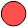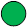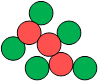### Sample Problemis an atom of element Xis an atom of element Yis an atom of element Z

Using the above information, how many X, Y, and Z molecules are in the compound shown below?Number of atoms of X

Number of atoms of Y

Number of atoms of Z

#### Solution

There are 5 green circles and 3 red circles so there are 5 atoms of element Z and 3 atoms of element X. Because there are no blue circles, the number of atoms of element Y is 0.